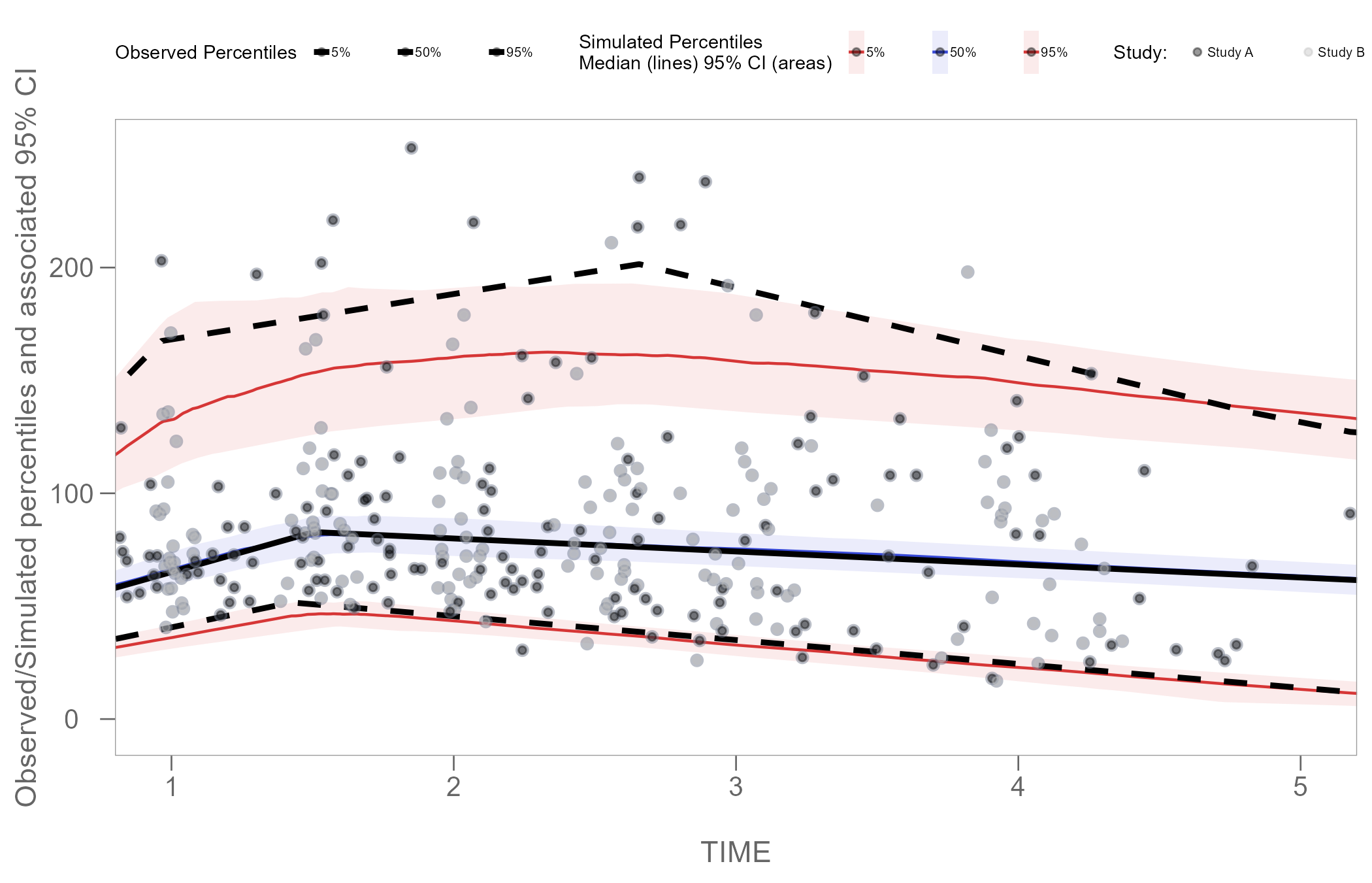## Overview

Certara.VPCResults uses functions from both the tidyvpc and ggplot2 packages to parameterize and plot Visual Predictive Checks (VPC). The resulting plot objects generated from the Shiny GUI are of class ggplot, which means we can use additional functions from the ggplot2 package to further customize our plot output inside RStudio.

### Tag Base VPC Plot

To begin, we will use the example obs_data and sim_data from the tidyvpc package.

First, we’ll need to subset our data by filtering MDV == 0, which removes rows where both DV == 0 and TIME == 0.

library(tidyvpc)

obs_data <- tidyvpc::obs_data[MDV == 0]
sim_data <- tidyvpc::sim_data[MDV == 0]

Next, we’ll launch the Shiny GUI using the function vpcResultsUI(), passing the obs_data and sim_data objects above to the observed and simulated arguments in the function vpcResultsUI().

library(Certara.VPCResults)

vpcResultsUI(observed = obs_data, simulated = sim_data)

The below images demonstrate how to tag a plot and download the R script to reproduce.

From the “Inputs” container, select the columns in the data to use for the x and y variables e.g., Time and DV.

Next, navigate to the “Controls” container and select from either binning or binless methods, then click “Plot”.

After generating the VPC plot and making any additional changes to the plot customization, click the blue circular button in the bottom right corner of the page, also known as the “Tag” button.

Once the plot has been “tagged” it will become available to view inside the “Tagged” tab, where you may preview the tidyvpc and ggplot2 code and download the R script to reproduce all tagged VPC plots from RStudio.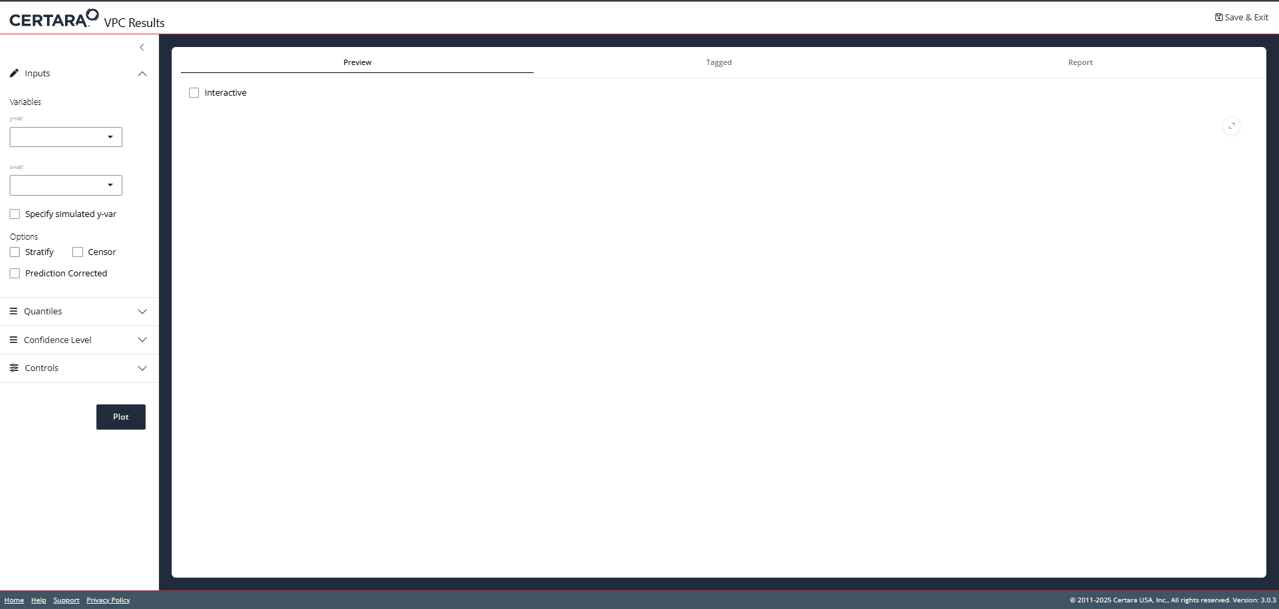The following R script my_vpc_script.R was downloaded from the “Tagged” tab in the Shiny GUI.

library(Certara.VPCResults)
library(ggplot2)
library(dplyr)
library(magrittr)
library(tidyvpc)

vpc <- observed(obs_data, x = TIME, y = DV) %>%
simulated(sim_data, y = DV) %>%
binless(optimize = TRUE, interval = c(0L, 7L)) %>%
vpcstats(qpred = c(0.05, 0.5, 0.95), conf.level = 0.95, quantile.type = 6)

vpcPlot <- ggplot(vpc$stats, aes(x = x)) + geom_ribbon(aes(ymin = lo, ymax = hi, fill = qname, col = qname, group = qname), alpha = 0.1, col = NA) + geom_line(aes(y = md, col = qname, group = qname)) + geom_line(aes(y = y, linetype = qname), size = 1) + geom_point(data = vpc$obs[!(blq | alq)], aes(x = x, y = y), color = "#757D8F", size = 2L, shape = 16, alpha = 0.5, show.legend = FALSE) +
scale_colour_manual(name = "Simulated Percentiles\nMedian (lines) 95% CI (areas)", breaks = c("q0.05", "q0.5", "q0.95"), values = c("#D63636", "#3648D6", "#D63636"), labels = c("5%", "50%", "95%")) +
scale_fill_manual(name = "Simulated Percentiles\nMedian (lines) 95% CI (areas)", breaks = c("q0.05", "q0.5", "q0.95"), values = c("#D63636", "#3648D6", "#D63636"), labels = c("5%", "50%", "95%")) +
scale_linetype_manual(name = "Observed Percentiles", breaks = c("q0.05", "q0.5", "q0.95"), values = c("dashed", "solid", "dashed"), labels = c("5%", "50%", "95%")) +
guides(fill = guide_legend(order = 2), colour = guide_legend(order = 2), linetype = guide_legend(order = 1)) +
ylab(sprintf("Observed/Simulated percentiles and associated %s%% CI", 100 * vpc$conf.level)) + xlab("\nTIME") + theme_certara() vpcPlot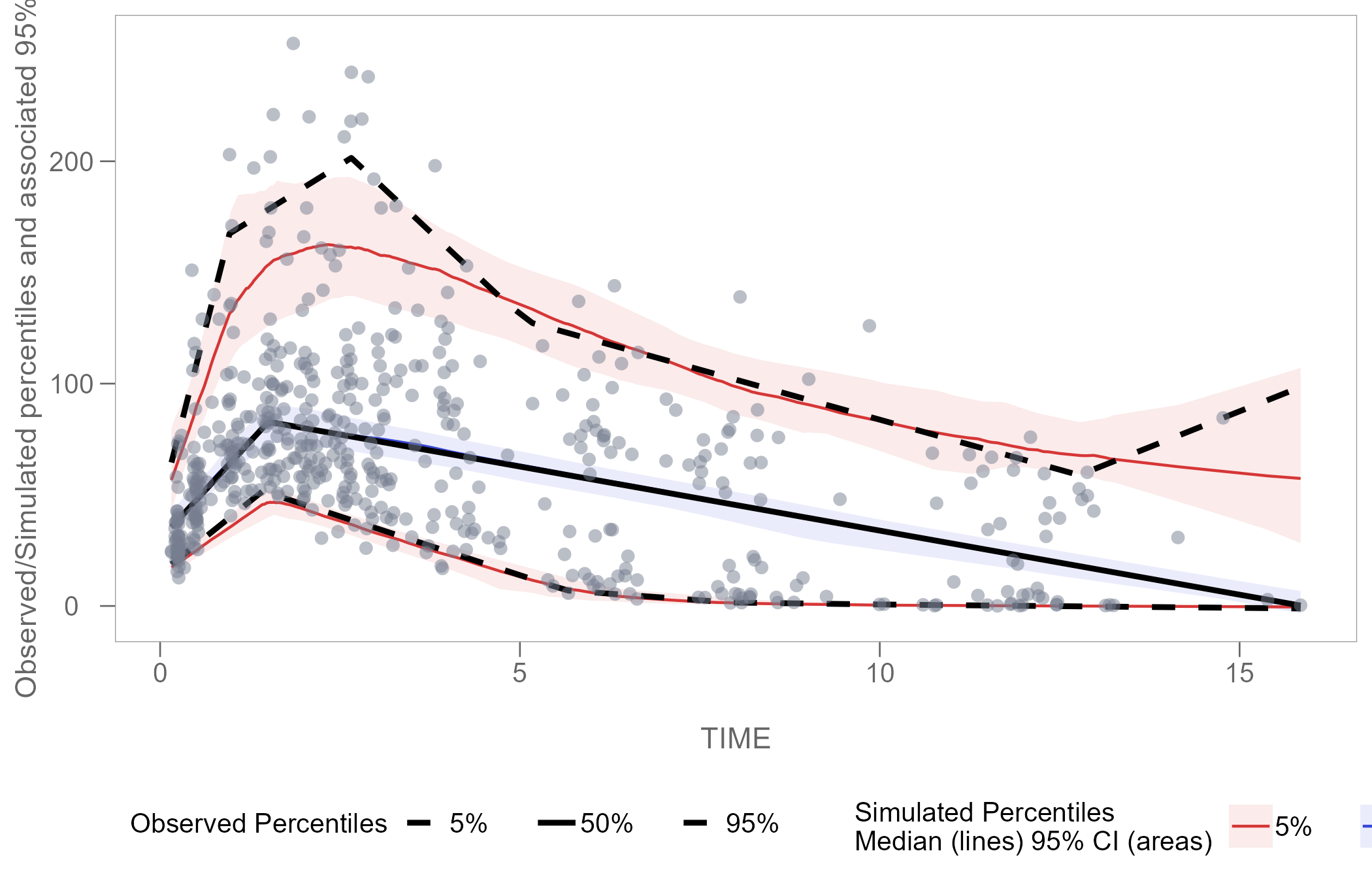## Plot Customization Now that we have our vpcPlot object (which is of class ggplot) in our local R environment, we can apply further plot customization using functions from ggplot2. ### Adjust Legend We can edit the size and spacing of text in the legend using the theme() argument. library(ggplot2) vpcPlot <- vpcPlot + theme(legend.position = "top", legend.title=element_text(size=8), legend.text=element_text(size=6), legend.key.width = unit(0.3, 'cm')) vpcPlot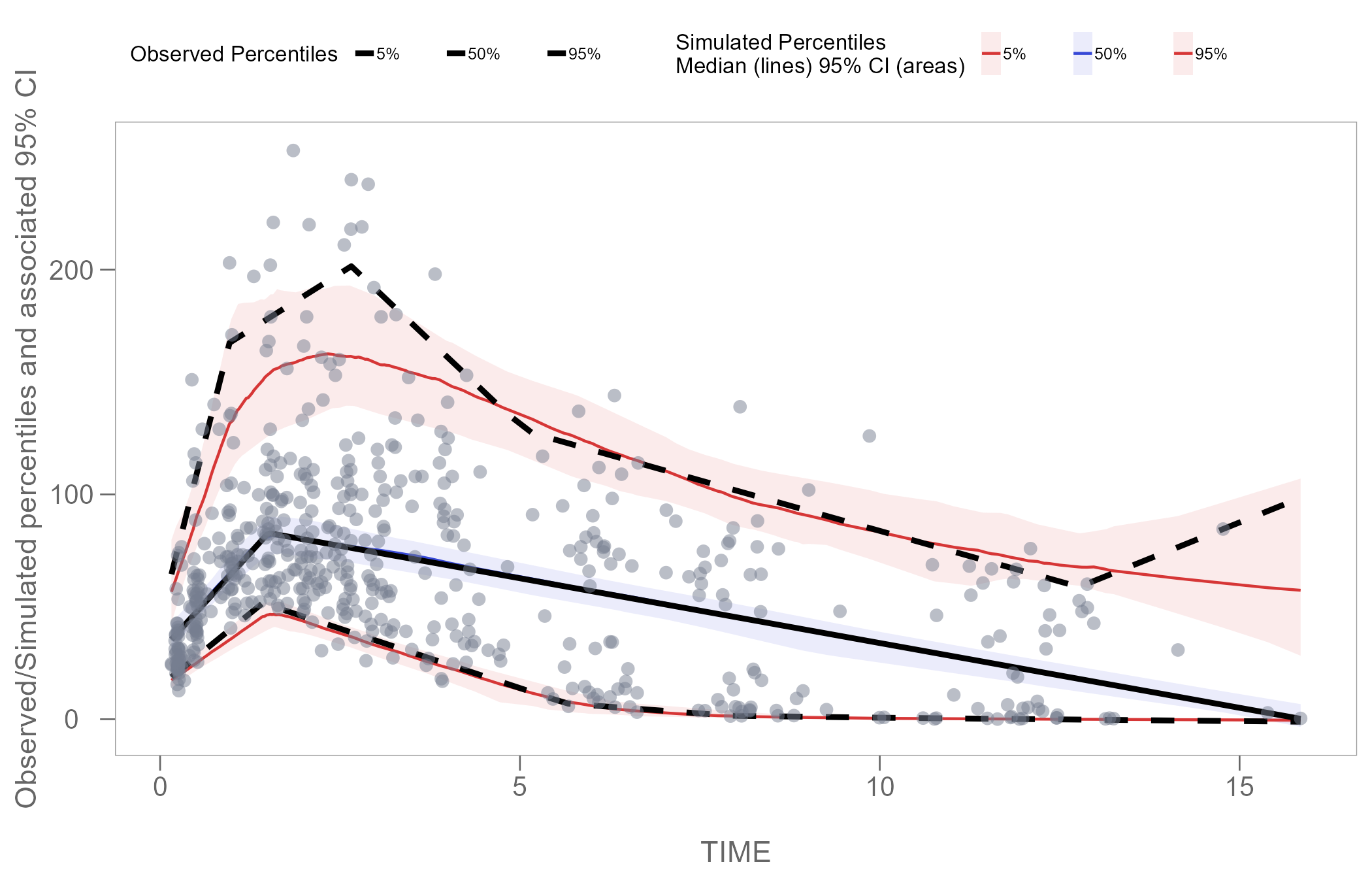### Zoom Plot Area Using the function coord_cartesian() from the ggplot2 package, we can zoom in on specific coordinates of interest in our plot. The example below shows how to set coordinates to display only time interval 1-5. vpcPlot <- vpcPlot + coord_cartesian(xlim = c(1, 5)) vpcPlot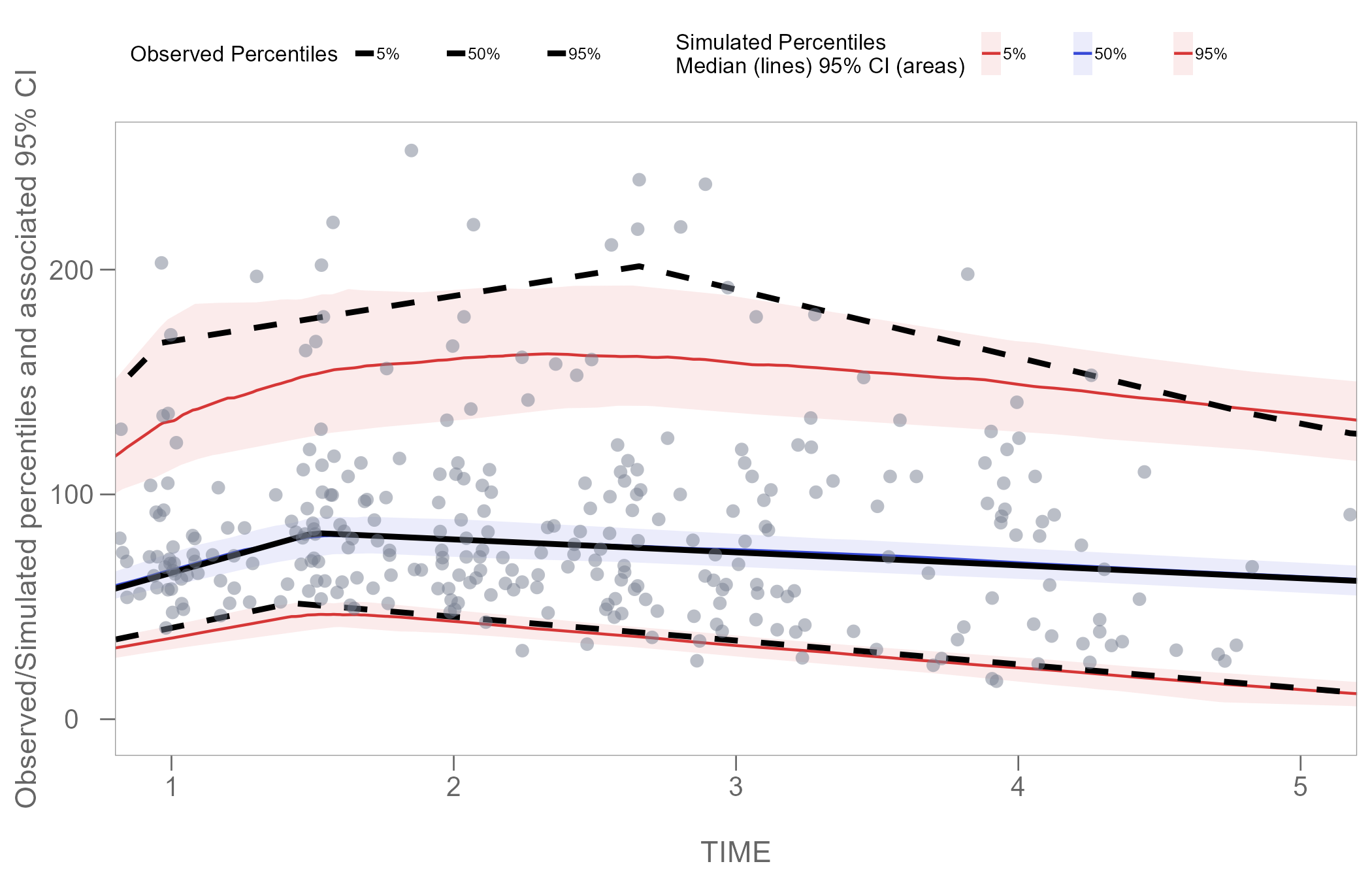Note: Windows users with default graphics device engine specified in RStudio may experience an issue where the fill of the geom_ribbon() in the plot disappears when combined with coord_cartesian(). The solution is to install the ragg package and set default graphics device engine in Rstudio to AGG. ### Color Points by Categorical Variable Instead of stratifying and faceting the resulting VPC plot by some categorical variable, we may choose to generate a single VPC plot and color the points to differentiate the unique values of the variable in the data. In the plot below, we will color points using the STUDY column in our obs_data. The STUDY column is of class character and has 2 unique values:  table(obs_data$STUDY)
#>
#> Study A Study B
#>     275     275

Using the code below, we will set the color of observations in Study A to black, and observations in Study B to grey. However, to achieve this, we must first add a new scale to our vpcPlot using functions from the package ggnewscale.

We will also edit the legend once again using the theme() function, to ensure that our new Study key displays correctly.

library(ggnewscale)

vpcPlot <- vpcPlot +
new_scale_colour() +
new_scale_fill() +
geom_point(data = vpc\$data,
aes(x = TIME, y = DV, colour = factor(STUDY)),
size = 1, alpha = 0.4, show.legend = T) +
scale_colour_manual(name = "Study: ",
values = c("black", "grey"),
labels = c("Study A", "Study B")) +
theme(legend.position = "top",
legend.title=element_text(size=7),
legend.text=element_text(size=5),
legend.key.width = unit(0.25, 'cm'),
legend.spacing.x = unit(0.025, 'cm'),
legend.justification = c(0, 0),
legend.margin = margin(c(5, 5, 5, 0)))

vpcPlot